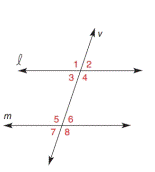Chapter 2.3, Problem 6EElementary Geometry For College St...

7th Edition
Alexander + 2 others
ISBN: 9781337614085

Solutions

Chapter
SectionElementary Geometry For College St...

7th Edition
Alexander + 2 others
ISBN: 9781337614085
Textbook Problem

In Exercises 1 to 6 , l and m are cut by transversal v . On the basis of the information given, determine whether l must be parallel to m .m ∠ 6 = 71.4 ° and m ∠ 7 = 71.4 °

To determine

To check:

The given statement.

Explanation

Given:

and m are cut by transversal v.

m6=71.4° and m7=71.4°

Approach:

Vertically opposite angles are congruent to each other.

Calculation:

The given statement is,

and m are cut by transversal v.

m6=71.4°

And,

m7=71

Still sussing out bartleby?

Check out a sample textbook solution.

See a sample solution

The Solution to Your Study Problems

Bartleby provides explanations to thousands of textbook problems written by our experts, many with advanced degrees!

Get Started

In Exercises 2340, find the indicated limit. 26. limx23x

Applied Calculus for the Managerial, Life, and Social Sciences: A Brief Approach

True or False: i + j is a unit vector in the direction of 5i + 5j.

Study Guide for Stewart's Multivariable Calculus, 8th

Explain the distinction between a hypothesis and a prediction.

Research Methods for the Behavioral Sciences (MindTap Course List)